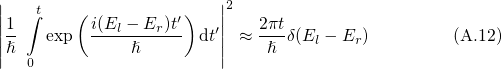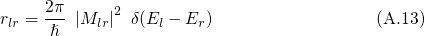A.1 Fermi’s Golden Rule

Fermi’s golden rule provides one way to calculate the transition rate between two certain quantum mechanically defined states. Due to its generality, it has various applications in the field of atomic, nuclear, and solid-state physics. In the case of NBTI, it is of most interest for charge transfer reactions and electron tunneling in particular. In the following, Fermi’s golden rule is derived for electron tunneling from the substrate into an oxide defect as illustrated in Fig. A.1.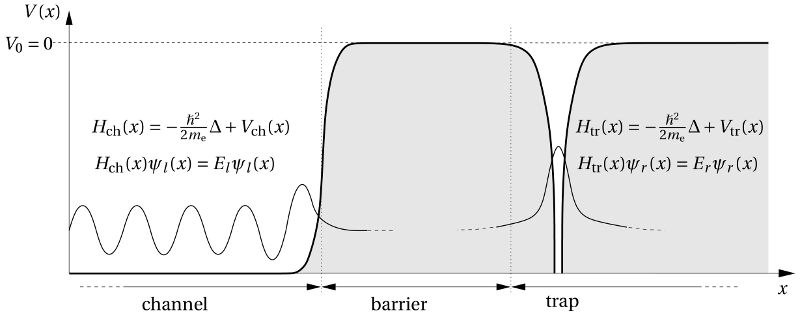Figure A.1: Schematic representation of the channel, the barrier, and the trap region. Interactions between the channel and the trap system are neglected to first order so that both systems are characterized by a separate Hamiltonian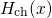and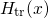, respectively. This means that the attractive trap potential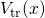is not accounted for in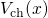so that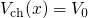in the trap region. Vice versa, the channel potentialis omitted in, which consequently takes the value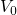in the channel region.

The system is divided into three separate regions, namely the channel, the insulator barrier, and the trap region. The electron wavefunctions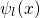and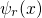extend into the classically forbidden barrier region. Their overlap actually leads to a mutual influence between the channel and the trap system. However, this influence is assumed to be negligible so that both systems can be treated independently to first order. This justifies the assumption that in a first approximation the channel and the trap system can be described by their own Hamiltonians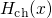and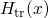. For the derivation of the tunneling rate, the Hamiltonian of the common system is taken as a starting point.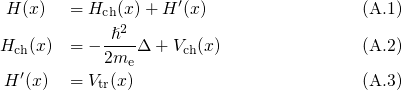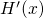is viewed as the time-dependent perturbation that triggers the scattering from the band states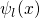into the trap states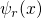. The solution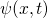of the common system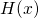can be written as a linear combination of the eigen wavefunctionsof the unperturbed system.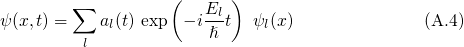This expansion of the wavefunction is inserted into the time-dependent Schrödinger equation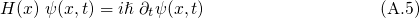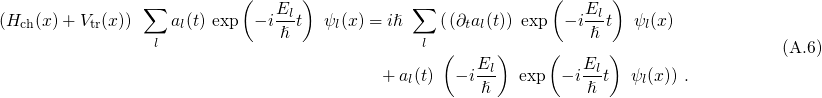Due to, the above equation simplifies to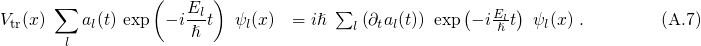Multiplying both sides by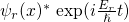from the left and integrating over space yields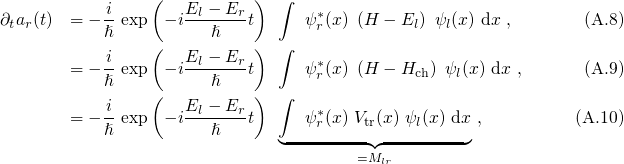where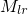is referred to as the matrix element.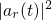gives the transition probabilitythat an electron initially located in the state, evolves into the final statesafter a time t. Therefore, it must be divided by the timein order to yield the transition rate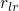.The integrand is sharply peaked at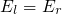and can be approximated as a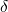-function.